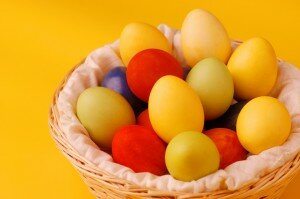# Easter Egg Hunt 100 and UnderEaster is the central feast and holiday in the Christian year representing Jesus’ Ascension.  It culminates the end of 40 days of fasting from the person’s choice.  Some people refrain from eating chocolate for 40 days until Easter weekend! Then they have an Easter Egg Hunt whereby one person hides chocolate eggs and the others have a fun treasure hunt to find the eggs.  For the Unity Spiritual Community, this year’s Easter’s theme is “Release Your Inner Splendor”. http://www.unity.org/publications/free-materials/lent-2012-release-your-inner-splendor

The following is a Fun Easter Egg Hunting Game for Practicing Addition, Subtraction, Multiplication and Division Under 100.  It can be played outdoors or indoors, depending on the weather and environment of the class.

Materials:

Organic Chocolate Eggs with foil covering

Marker

Pencil

Paper

Instructions:

Find a box of Organic Chocolate Easter Eggs.

Unwrap each egg but save the foil covering.

1. Make up a set of Math Problems to Solve, including addition, subtraction, multiplication and division.  At the end of this article, we have included a sample.

2. Wrap each Egg with a problem written on a small piece of paper, and then rewrap the eggs with the foil.  With a marker, number the eggs by groups.

3. Divide Learners into groups of 3, and Assign each group a number.  (In this example, there are 21 students. The class would be divided into 7 groups of 3, and there would be 7 groups of Math Problems).

4.Hide the eggs outdoors and/or indoors, weather permitting.

5. Each group has paper and pencil.  Each group hunts for eggs with their numbers and solves the math problems. As they find the eggs, each group respectively puts them in their basket. Each Learner Individually solves the problem, by first writing the problem on his/her paper and then following with the answer.

6. As each is finished, they bring their sheets to Teacher for checking. If they have any answers wrong, they must redo their answer. When all answers are correct, they can eat the chocolate, and help other groups.

Remember to recycle all the foil and paper wrappings!

http://kidsblogs.nationalgeographic.com/greenscene/2012/03/recycled-kisses.html

http://www.bayroberts.com/green/reduce.htm

Here are some more of our Fun Learning Math Games:

http://www.math-lessons.ca/activities/index.html

http://www.math-lessons.ca/review/math-review4.html

http://www.math-lessons.ca/timestables/times-tables.html

Sample Math Problems:

Group 1:

84 + 11 = 95

88 – 35 = 53

24 */* 3 = 8

8 x 6 = 48

14 */* 7 = 2

88 – 31 = 57

4  x 18 = 72

75 */* 3 = 25

25 – 5 = 20

Group 2:

88 + 12 = 100

25 x 3 = 75

18 */* 2 = 9

22  x  4 = 88

21 */* 3 = 7

55 –11 = 44

23 x 4 = 92

24 */* 12 = 2

45 + 33 = 78

Group 3:

88 – 3 = 85

13 x 4 = 52

100 */*25 = 4

44 x 2 = 88

13 – 1 = 12

44 */* 4 = 11

77 */* 11 = 7

3 x 9 = 27

52 – 23 = 29

Group 4:

44 – 11 = 33

23 x 4 = 92

14 */* 2 = 7

28 + 14 = 42

55 */* 11 = 5

88 – 13 = 75

34 – 17 = 17

22 x 4 = 88

14 + 17 = 31

Group 5:

14 + 5 = 19

33 – 12 = 21

22 x 5 = 77

89 – 17 = 72

34 +55 = 89

33 */* 3 = 11

22 x 4 = 88

100 */* 4 = 25

21 x 4 = 84

Group 6:

19 + 24 =43

47 – 17 =30

88 */* 11 = 8

24 x 3 = 72

90 */* 10 = 9

55 – 23 = 32

17 x 5 = 85

9 */* 3 = 3

100 – 25 = 75

Group 7:

18 */* 9 = 2

7 x 7 = 49

21 x 4 = 84

88 – 55 = 33

21 + 4 = 25

99 */* 3 = 33

22 x 1 = 22

35 – 8 = 27

77 – 22 = 55